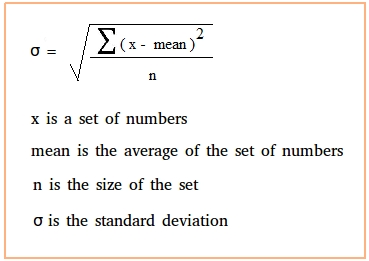# Standard Deviation Calculator

## Why use an online standard deviation calculator?

MYcalcu’s standard deviation calculator uses your data set and shows the work required for the calculations.

It helps you to find the standard deviation, variance, count of data points n, mean, and sum of squares.

Standard deviation is a statistical measure of diversity or variability in a data set.

A low standard deviation indicates that data points are generally close to the mean or the average value. A high standard deviation indicates greater variability in data points or higher dispersion from the mean.

## Standard Deviation Calculator Results

 Standard Deviation variance Mean Total Numbers Sum of Numbers

## How does it work?

The formula for standard deviation is:## How to use a standard deviation calculator?

Enter your data set with commas, lines, and spaces separated from each other.

You can also copy and paste your data from a spreadsheet or a document.